# 4.2 Analytical solution methods for the two-dimensional Helmholtz equation

A solution of (4.13) with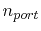excitation ports, defined between the cover and the bottom plane (Figure 4.2), relates the port voltages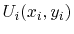on the ports with index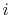to the currents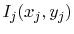on the ports with index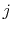by the impedance matrix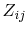. The coordinates of port i and port j are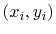and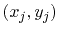respectively.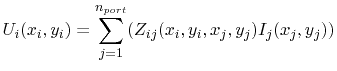with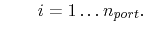(4.17)

An analytical solution for rectangular parallel planes with four open edges depicted in Figure 4.2 was presented by . In this solution, the coefficients of the impedance matrix in (4.17) are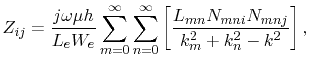(4.18)

with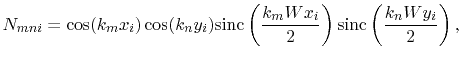(4.19)(4.20)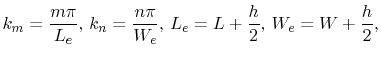(4.21)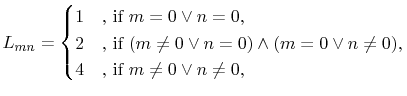(4.22)

and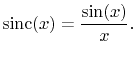(4.23)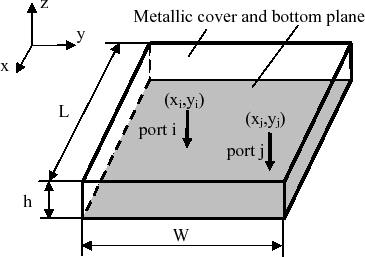In (4.18)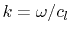denotes the wave number, with the speed of light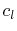.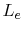and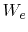are the effective plane length and width, respectively. These effective dimensions consider the fringing fields at the cavity edges according to .  also provides an analytical solution for equilateral triangular parallel planes with three open edges. Chapter 7 of this work presents an analytical solution for rectangular parallel planes with one open and three closed edges. This is a powerful solution for predesign investigations of the radiated emissions from the slot of a slim cubical enclosure, because discussion of the bias functions of the model provides direct information about the influence of the source position on the emission level.
The analytical method of  enables the calculation of parallel plane cavities with fairly arbitrary shapes by connecting rectangular or equilateral triangular parallel-plane segments. This method is illustrated in Figure 4.3.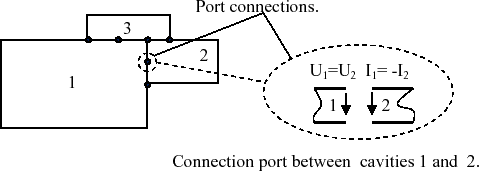C. Poschalko: The Simulation of Emission from Printed Circuit Boards under a Metallic Cover